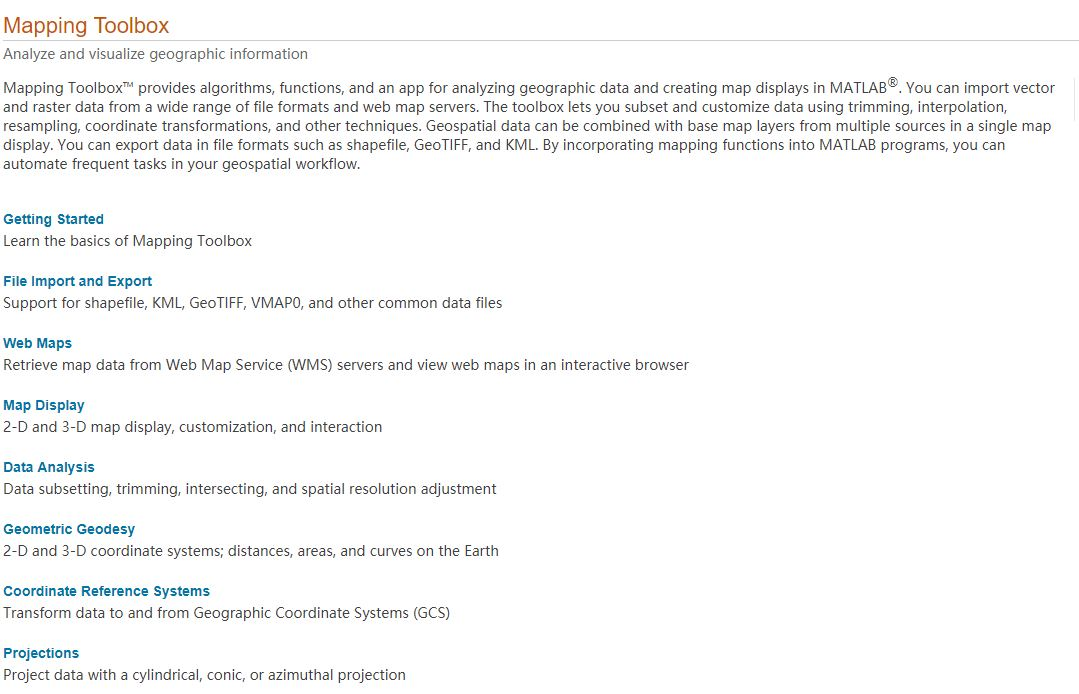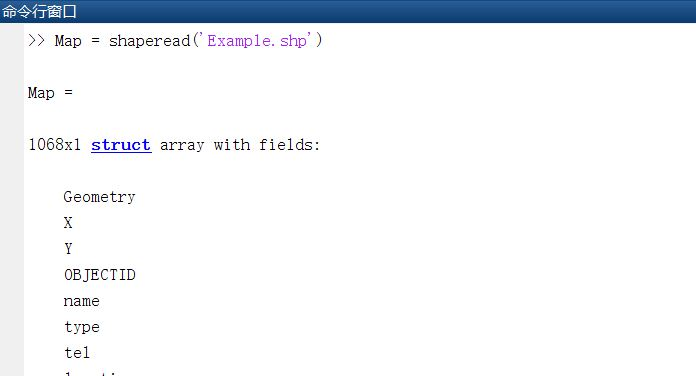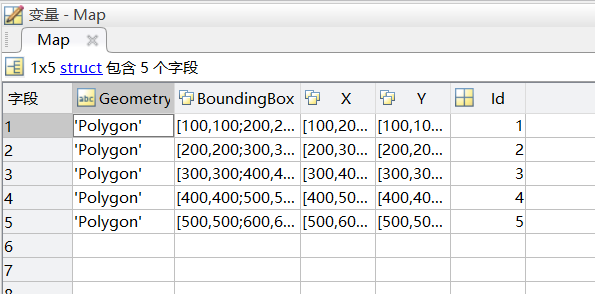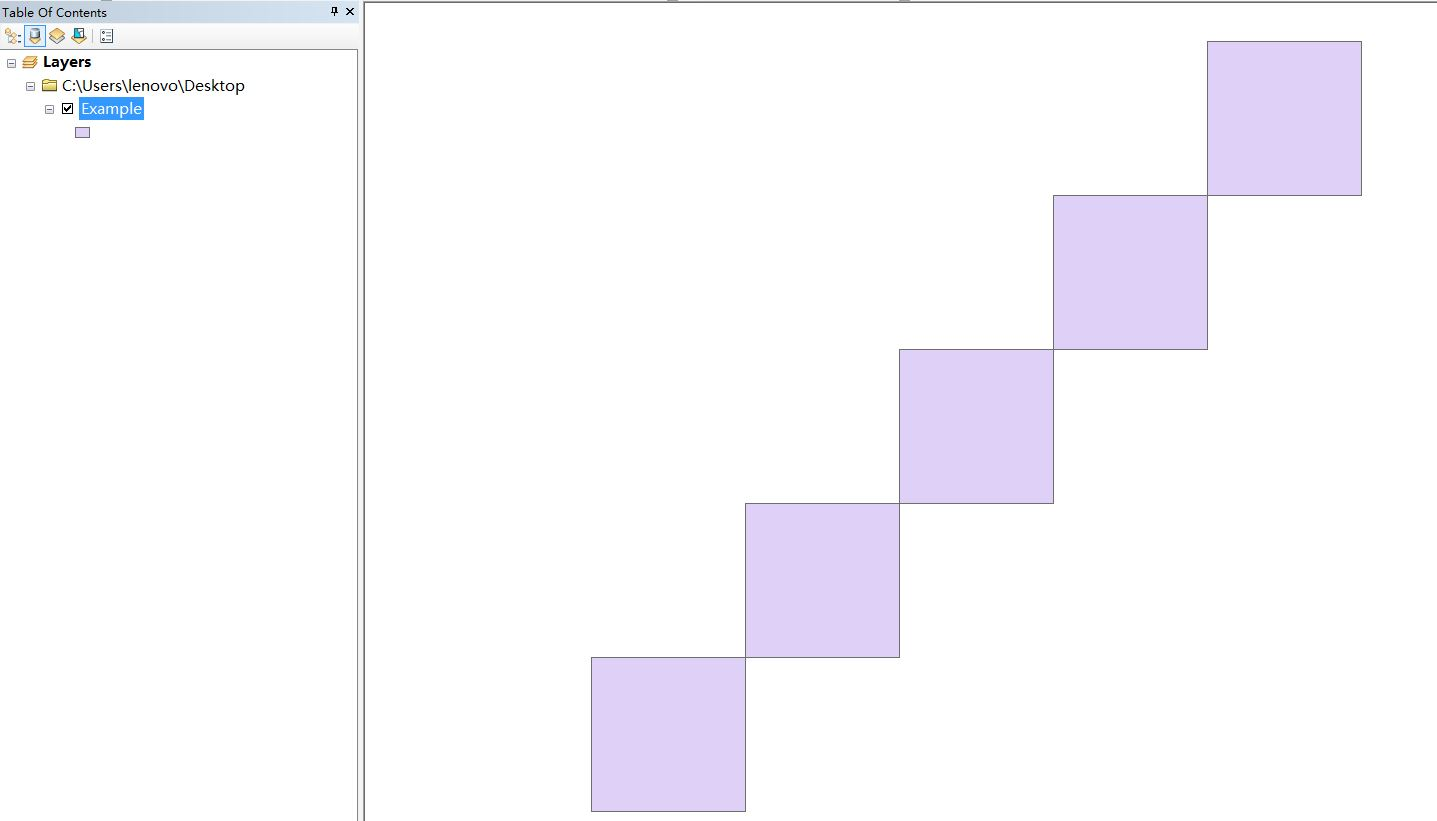• 4. 3.2 结构数组的操作MATLAB中专门用于对结构数组的操作的函数并不多，通过 help datatypes获取数据类型列表，可以看到其中的结构数据类型的有关的函数，主要如表4.3.1所示。表4.3.1 结构数组的操作函数函数名 功能...
4. 3.2 结构数组的操作MATLAB中专门用于对结构数组的操作的函数并不多，通过 help datatypes获取数据类型列表，可以看到其中的结构数据类型的有关的函数，主要如表4.3.1所示。表4.3.1 结构数组的操作函数函数名   功能描述   函数名   功能描述deal   把输入处理成输出   fieldnames   获取结构的字段名getfield   获取结构中指定字段的值   rmfield   删除结构的字段(不是字段内容)setfield   设置结构数组中指定的字段的值   struct   创建结构数组struct2cell   结构数组转化成元胞数组   isfield   判断是否存在该字段isstruct   判断某变量是否是结构类型下面举一些具体的例子说明如果对结构数组加以操作。【例4.3.2-1】 本例目的：一，演示函数fieldnames , getfield , setfield的使用方法；二，让读者感受到结构数组对应用工具包的影响；三，演示struct函数把“对象”转换为结构的应用。本例为获得一个演练的结构，借助Toolbox control 工具包中的tf函数，先产生一个用传递函数描写的LTI线性时不变2输入2输出系统 。(1)产生2输入2输出系统的传递函数阵“对象”Stf=tf({3,2;[4 1],1},{[1 3 2],[1 1 1];[1 2 2 1],[1 0]})Transfer function from input 1 to output...3#1: -------------s^2 + 3 s + 24 s + 1#2: ---------------------s^3 + 2 s^2 + 2 s + 1Transfer function from input 2 to output...2#1: -----------s^2 + s + 11#2: -s(2)为本例演示，把上述的LTI对象Stf转换为结构SSTF=struct(Stf)       % 把对象转换成结构，并显示结构的组成SSTF =num: {2x2 cell}den: {2x2 cell}Variable: 's'lti: [1x1 lti](3)获得结构数组SSTF的域名FN=fieldnames(SSTF) % 获得域名元胞数组FNclass(FN)     % 检查FN的类别FN ='num''den''Variable''lti'ans =cell(4)获取SSTF.den(2,1)域的内容FC=getfield(SSTF,'den',{2,1})     % 相当于FC=SSFT.den(2,1)FC{1}                   % 与celldisp(FC)的作用大致相当poly2str(FC{1},'s'),         % 为了把多项式显示成习惯的形式FC =[1x4 double]ans =1 2 2 1ans =s^3 + 2 s^2 + 2 s + 1(5)重新设置SSTF.num(2,1)域的内容SSTF.num{2,1}                 % 显示原始情况SSTF=setfield(SSTF,'num',{2,1},{[1 3 1]}); %   注意“花括号”的使用SSTF.num{2,1}                     % 显示被重新设置后的情况ans =0 0 4 1ans =1 3 1【例4.3.2-2】本例演示结构数组SSTF的扩充和收缩。(本例以例4.3.2-1的运行为基础。)(1)原结构是一个“单结构”size(SSTF)ans =1 1(2)演示结构的扩充SSTF(2,2)=struct(tf(1,[1 1]))     % 把1/(s+1)放在第2行第2列结构中size(SSTF)SSTF =2x2 struct array with fields:numdenVariableltians =2 2(3)演示结构数组的收缩：删除结构数组的第1行SSTF(1,=[]                   % 收缩成为 的结构S22n=SSTF(1,2).num,S22d=SSTF(1,2).den   % 取出第2结构num域和den域的内容printsys(S22n{1},S22d{1})           % 显示成习惯的表达形式SSTF =1x2 struct array with fields:numdenVariableltiS22n =[1x2 double]S22d =[1x2 double]num/den =1-----s + 1【例4.3.2-3】对结构数组进行域的增添和删减操作。(1)创建结构数组clear,for k=1:10;department(k).number=['No.',int2str(k)];enddepartmentdepartment =1x10 struct array with fields:number(2)增添域：在数组中任何一个结构上进行的域增添操作，其影响遍及整个结构数组department(1).teacher=40;department(1).student=300;department(1).PC_computer=40;departmentdepartment =1x10 struct array with fields:numberteacherstudentPC_computer(3)增添子域的操作只影响被操作的那个具体结构，而不是影响整个结构数组department(2).teacher.male=35;department(2).teacher.female=13;D2T=department(2).teacher     % 第2结构teacher域包含两个子域D1T=department(1).teacher     % 第1结构teacher域仅是一个数D2T =male: 35female: 13D1T =40(4)删除子域的操作也只影响被操作的那个具体结构department(2).teacher=rmfield(department(2).teacher,'male');department(2).teacherans =female: 13(5)删除域的操作是对整个结构数组实施的department=rmfield(department,'student')   % 删除一个域department =1x10 struct array with fields:numberteacherPC_computerdepartment=rmfield(department,{'teacher';'PC_computer'})% 删除2个域department =1x10 struct array with fields:number【例4.3.2-4】数值运算操作和函数在结构域上的作用。n_ex = 5; % 结构数组的长度for k = 1:n_ex,   % 创建1×5结构数组ex(k).f = (k-1)*n_ex + [1:5];end;ex                           % 显示结构数组的结构ex =1x5 struct array with fields:f%显示结构数组的域中内容disp([blanks(10) '结构域中内容'])for k=1:n_ex,disp(ex(k).f),end结构域中内容1 2 3 4 56 7 8 9 1011 12 13 14 1516 17 18 19 2021 22 23 24 25class(ex(1).f)                   % 检查域中内容的类型ans =double% 对各结构域中数值数组相应位置的数据相加求和sum_f=zeros(1,5)for k=1:n_ex,sum_f=sum_f+ex(k).f;end,sum_fsum_f =55 60 65 70 75% 对结构数组域中各元素分别求平方根disp([blanks(20) 'ex.f的平方根值'])for k=1:n_ex,disp(sqrt(ex(k).f)),endex.f的平方根值1.0000 1.4142 1.7321 2.0000 2.23612.4495 2.6458 2.8284 3.0000 3.16233.3166 3.4641 3.6056 3.7417 3.87304.0000 4.1231 4.2426 4.3589 4.47214.5826 4.6904 4.7958 4.8990 5.0000【例4.3.2-5】   指令struct2cell和cell2struct的使用。(1)创建“带2个域的 结构数组”for k=1:5,ex(k).s=['No.' int2str(k)];ex(k).f=(k-1)*5+[1:5];end(2)显示结构数组的内容fprintf('%s\n','ex.s域的内容 ');fprintf('%s\',blanks(4))for k=1:5;fprintf('%s\\',[ex(k).s blanks(1)]);endfprintf('%s\n',blanks(1)),fprintf('%s\n','ex.f域的内容 ')for k=1:5;disp(ex(k).f);end     %显示ex.f域内容ex.s域的内容No.1 \No.2 \No.3 \No.4 \No.5 \ex.f域的内容1 2 3 4 56 7 8 9 1011 12 13 14 1516 17 18 19 2021 22 23 24 25(3)把ex结构数组转换为元胞数组C_ex=struct2cell(ex); % 带2个域的(1×5)结构数组转换为(2×1×5)元胞数组size(C_ex)fprintf('%s\',[C_ex{1,1,1},blanks(3)])   % 显示C_ex第1页第1行第1列内容fprintf('%5g\',C_ex{2,1,1})         % 显示C_ex第2页第1行第1列内容ans =2 1 5No.1 1 2 3 4 5(4)把元胞数组转换为结构数组之一FS={'S_char';'F_num'};        % 用元胞数组预建域名字符串EX1=cell2struct(C_ex,FS,1)      % 元胞数组向结构数组转换EX1 =1x5 struct array with fields:S_charF_numricEX1(1)                   % 观察新结构EX1第一结构的情况ans =S_char: 'No.1'F_numric: [1 2 3 4 5](5)把元胞数组转换为结构数组之二EX2=cell2struct(C_ex,'xx',2)EX2 =2x5 struct array with fields:xx(6)把元胞数组转换为结构数组之三YY=strvcat('y1','y2','y3','y4','y5');EX3=cell2struct(C_ex,YY,3)EX3 =2x1 struct array with fields:y1y2y3y4y5EX3(1)                   % 观察第一结构情况ans =y1: 'No.1'y2: 'No.2'y3: 'No.3'y4: 'No.4'y5: 'No.5'EX3(2)                   % 观察第二结构情况ans =y1: [1 2 3 4 5]y2: [6 7 8 9 10]y3: [11 12 13 14 15]y4: [16 17 18 19 20]y5: [21 22 23 24 25]【例4.3.2-6】   带子域的结构数组转换为元胞数组。本例中的ex结构数组由例4.2.2-5生成，然后再运行以下程序。ex(1,1).s                 % 原结构ex(1,1).s中的内容ans =No.1% 增设子域，并把ex结构数组扩充为(3×5)。ex(1,1).s.sub='SUB 1';         % 原ex(1,1).s中的字符串将因本指令而消失ex(3,1).s.sub='SUB 3';ex(3,1).s.num=1/3;ex(1,1).s                 % 经新赋值后，ex(1,1).s中的内容ans =sub: 'SUB 1'ex(3,1).s                 % 经新赋值后，ex(3,1).s中的内容ans =sub: 'SUB 3'num: 0.3333C_ex_sub=struct2cell(ex)       % 把结构转换为元胞数组C_ex_sub(:,:,1) =[1x1 struct] [] [1x1 struct][1x5 double] [] []C_ex_sub(:,:,2) ='No.2' [] [][1x5 double] [] []C_ex_sub(:,:,3) ='No.3' [] [][1x5 double] [] []C_ex_sub(:,:,4) ='No.4' [] [][1x5 double] [] []C_ex_sub(:,:,5) ='No.5' [] [][1x5 double] [] []size(C_ex_sub)             % 观察新元胞数组的大小ans =2 3 5C_ex_sub{1,1,1}             % 观察第一元胞中的内容ans =sub: 'SUB 1'C_ex_sub{1,3,1}             % 观察(1,3,1)元胞中的内容ans =sub: 'SUB 3'num: 0.3333
展开全文• matlab开发-struct2csv。使用列标题将结构输出到.csv文件
• ## matlab 中的 struct(2)

千次阅读 2010-07-09 14:28:00
matlab 结构(struct)数组(转） MATLAB学习2009-07-28 09:28:51阅读366评论0字号：大中小 MATLAB中专门用于对结构数组的操作的函数并不多，通过 help datatypes获取数据类型列表，可以看到其中的结构数据...
matlab 结构(struct)数组(转）
MATLAB学习 2009-07-28 09:28:51 阅读366 评论0 字号：大中小

MATLAB中专门用于对结构数组的操作的函数并不多，通过 help datatypes获取数据类型列表，可以看到其中的结构数据类型的有关的函数，主要如表4.3.1所示。
表4.3.1 结构数组的操作函数
函数名   功能描述   函数名   功能描述
deal   把输入处理成输出   fieldnames   获取结构的字段名
getfield   获取结构中指定字段的值   rmfield   删除结构的字段(不是字段内容)
setfield   设置结构数组中指定的字段的值   struct   创建结构数组
struct2cell   结构数组转化成元胞数组   isfield   判断是否存在该字段
isstruct   判断某变量是否是结构类型
下面举一些具体的例子说明如果对结构数组加以操作。
【例4.3.2-1】 本例目的：一，演示函数fieldnames , getfield , setfield的使用方法；二，让读者感受到结构数组对应用工具包的影响；三，演示struct函数把“对象”转换为结构的应用。本例为获得一个演练的结构，借助Toolbox control 工具包中的tf函数，先产生一个用传递函数描写的LTI线性时不变2输入2输出系统 。
（1）产生2输入2输出系统的传递函数阵“对象”
Stf=tf({3,2;[4 1],1},{[1 3 2],[1 1 1];[1 2 2 1],[1 0]})
Transfer function from input 1 to output...
3
#1: -------------
s^2 + 3 s + 2
4 s + 1
#2: ---------------------
s^3 + 2 s^2 + 2 s + 1
Transfer function from input 2 to output...
2
#1: -----------
s^2 + s + 1
1
#2: -
s
（2）为本例演示，把上述的LTI对象Stf转换为结构
SSTF=struct(Stf)       % 把对象转换成结构，并显示结构的组成
SSTF =
num: {2x2 cell}
den: {2x2 cell}
Variable: 's'
lti: [1x1 lti]
（3）获得结构数组SSTF的域名
FN=fieldnames(SSTF) % 获得域名元胞数组FN
class(FN)     % 检查FN的类别
FN =
'num'
'den'
'Variable'
'lti'
ans =
cell
（4）获取SSTF.den(2,1)域的内容
FC=getfield(SSTF,'den',{2,1})     % 相当于FC=SSFT.den(2,1)
FC{1}                   % 与celldisp(FC)的作用大致相当
poly2str(FC{1},'s'),         % 为了把多项式显示成习惯的形式
FC =
[1x4 double]
ans =
1 2 2 1
ans =
s^3 + 2 s^2 + 2 s + 1
（5）重新设置SSTF.num(2,1)域的内容
SSTF.num{2,1}                 % 显示原始情况
SSTF=setfield(SSTF,'num',{2,1},{[1 3 1]}); %   注意“花括号”的使用
SSTF.num{2,1}                     % 显示被重新设置后的情况
ans =
0 0 4 1
ans =
1 3 1
【例4.3.2-2】本例演示结构数组SSTF的扩充和收缩。（本例以例4.3.2-1的运行为基础。）
（1）原结构是一个“单结构”
size(SSTF)
ans =
1 1
（2）演示结构的扩充
SSTF(2,2)=struct(tf(1,[1 1]))     % 把1/(s+1)放在第2行第2列结构中
size(SSTF)
SSTF =
2x2 struct array with fields:
num
den
Variable
lti
ans =
2 2
（3）演示结构数组的收缩：删除结构数组的第1行
SSTF(1,)=[]                   % 收缩成为 的结构
S22n=SSTF(1,2).num,S22d=SSTF(1,2).den   % 取出第2结构num域和den域的内容
printsys(S22n{1},S22d{1})           % 显示成习惯的表达形式
SSTF =
1x2 struct array with fields:
num
den
Variable
lti
S22n =
[1x2 double]
S22d =
[1x2 double]
num/den =
1
-----
s + 1
【例4.3.2-3】对结构数组进行域的增添和删减操作。
（1）创建结构数组
clear,for k=1:10;department(k).number=['No.',int2str(k)];end
department
department =
1x10 struct array with fields:
number
（2）增添域：在数组中任何一个结构上进行的域增添操作，其影响遍及整个结构数组
department(1).teacher=40;department(1).student=300;
department(1).PC_computer=40;
department
department =
1x10 struct array with fields:
number
teacher
student
PC_computer
（3）增添子域的操作只影响被操作的那个具体结构，而不是影响整个结构数组
department(2).teacher.male=35;department(2).teacher.female=13;
D2T=department(2).teacher     % 第2结构teacher域包含两个子域
D1T=department(1).teacher     % 第1结构teacher域仅是一个数
D2T =
male: 35
female: 13
D1T =
40
（4）删除子域的操作也只影响被操作的那个具体结构
department(2).teacher=rmfield(department(2).teacher,'male');
department(2).teacher
ans =
female: 13
（5）删除域的操作是对整个结构数组实施的
department=rmfield(department,'student')   % 删除一个域
department =
1x10 struct array with fields:
number
teacher
PC_computer
department=rmfield(department,{'teacher';'PC_computer'})% 删除2个域
department =
1x10 struct array with fields:
number
【例4.3.2-4】数值运算操作和函数在结构域上的作用。
n_ex = 5; % 结构数组的长度
for k = 1:n_ex,   % 创建1×5结构数组
ex(k).f = (k-1)*n_ex + [1:5];
end;
ex                           % 显示结构数组的结构
ex =
1x5 struct array with fields:
f
%显示结构数组的域中内容
disp([blanks(10) '结构域中内容'])
for k=1:n_ex,disp(ex(k).f),end
结构域中内容
1 2 3 4 5
6 7 8 9 10
11 12 13 14 15
16 17 18 19 20
21 22 23 24 25
class(ex(1).f)                   % 检查域中内容的类型
ans =
double
% 对各结构域中数值数组相应位置的数据相加求和
sum_f=zeros(1,5)
for k=1:n_ex,sum_f=sum_f+ex(k).f;end,sum_f
sum_f =
55 60 65 70 75
% 对结构数组域中各元素分别求平方根
disp([blanks(20) 'ex.f的平方根值'])
for k=1:n_ex,
disp(sqrt(ex(k).f)),
end
ex.f的平方根值
1.0000 1.4142 1.7321 2.0000 2.2361
2.4495 2.6458 2.8284 3.0000 3.1623
3.3166 3.4641 3.6056 3.7417 3.8730
4.0000 4.1231 4.2426 4.3589 4.4721
4.5826 4.6904 4.7958 4.8990 5.0000
【例4.3.2-5】   指令struct2cell和cell2struct的使用。
（1）创建“带2个域的 结构数组”
for k=1:5,
ex(k).s=['No.' int2str(k)];
ex(k).f=(k-1)*5+[1:5];
end
（2）显示结构数组的内容
fprintf('%s/n','ex.s域的内容 ');fprintf('%s/',blanks(4))
for k=1:5;fprintf('%s//',[ex(k).s blanks(1)]);end
fprintf('%s/n',blanks(1)),fprintf('%s/n','ex.f域的内容 ')
for k=1:5;disp(ex(k).f);end     %显示ex.f域内容
ex.s域的内容
No.1 /No.2 /No.3 /No.4 /No.5 /
ex.f域的内容
1 2 3 4 5
6 7 8 9 10
11 12 13 14 15
16 17 18 19 20
21 22 23 24 25
（3）把ex结构数组转换为元胞数组
C_ex=struct2cell(ex); % 带2个域的（1×5）结构数组转换为（2×1×5）元胞数组
size(C_ex)
fprintf('%s/',[C_ex{1,1,1},blanks(3)])   % 显示C_ex第1页第1行第1列内容
fprintf('%5g/',C_ex{2,1,1})         % 显示C_ex第2页第1行第1列内容
ans =
2 1 5
No.1 1 2 3 4 5
（4）把元胞数组转换为结构数组之一
FS={'S_char';'F_num'};        % 用元胞数组预建域名字符串
EX1=cell2struct(C_ex,FS,1)      % 元胞数组向结构数组转换
EX1 =
1x5 struct array with fields:
S_char
F_numric
EX1(1)                   % 观察新结构EX1第一结构的情况
ans =
S_char: 'No.1'
F_numric: [1 2 3 4 5]
（5）把元胞数组转换为结构数组之二
EX2=cell2struct(C_ex,'xx',2)
EX2 =
2x5 struct array with fields:
xx
（6）把元胞数组转换为结构数组之三
YY=strvcat('y1','y2','y3','y4','y5');EX3=cell2struct(C_ex,YY,3)
EX3 =
2x1 struct array with fields:
y1
y2
y3
y4
y5
EX3(1)                   % 观察第一结构情况
ans =
y1: 'No.1'
y2: 'No.2'
y3: 'No.3'
y4: 'No.4'
y5: 'No.5'
EX3(2)                   % 观察第二结构情况
ans =
y1: [1 2 3 4 5]
y2: [6 7 8 9 10]
y3: [11 12 13 14 15]
y4: [16 17 18 19 20]
y5: [21 22 23 24 25]
【例4.3.2-6】   带子域的结构数组转换为元胞数组。本例中的ex结构数组由例4.2.2-5生成，然后再运行以下程序。
ex(1,1).s                 % 原结构ex(1,1).s中的内容
ans =
No.1
% 增设子域，并把ex结构数组扩充为（3×5）。
ex(1,1).s.sub='SUB 1';         % 原ex(1,1).s中的字符串将因本指令而消失
ex(3,1).s.sub='SUB 3';
ex(3,1).s.num=1/3;
ex(1,1).s                 % 经新赋值后，ex(1,1).s中的内容
ans =
sub: 'SUB 1'
ex(3,1).s                 % 经新赋值后，ex(3,1).s中的内容
ans =
sub: 'SUB 3'
num: 0.3333
C_ex_sub=struct2cell(ex)       % 把结构转换为元胞数组
C_ex_sub(:,:,1) =
[1x1 struct] [] [1x1 struct]
[1x5 double] [] []
C_ex_sub(:,:,2) =
'No.2' [] []
[1x5 double] [] []
C_ex_sub(:,:,3) =
'No.3' [] []
[1x5 double] [] []
C_ex_sub(:,:,4) =
'No.4' [] []
[1x5 double] [] []
C_ex_sub(:,:,5) =
'No.5' [] []
[1x5 double] [] []
size(C_ex_sub)             % 观察新元胞数组的大小
ans =
2 3 5
C_ex_sub{1,1,1}             % 观察第一元胞中的内容
ans =
sub: 'SUB 1'
C_ex_sub{1,3,1}             % 观察(1,3,1)元胞中的内容
ans =
sub: 'SUB 3'
num: 0.3333

展开全文应用工具 function c
• 该代码是一个matlab工具包，用于完成机器学习中支持向量机的结构化输出支持向量机
• 问题： 如何获取一个未知的struct的所有field 函数：fieldnames test.a=1 test.b=2 fieldnames(test) 输出 ans = 2×1 cell array 'a' 'b'
问题：
如何获取一个未知的struct的所有field
函数：fieldnames
test.a=1
test.b=2
fieldnames(test)

输出
ans =

2×1 cell array

'a'
'b'




展开全文field
• * 数学软件 Matlab 文件操作 优选文档 Matlab数据类型 Matlab 数据类型的最大特点是每一种类型都是以数组为基础Matlab...cell单元型 struct结构 优选文档 * Matlab数据类型 数组 Matlab7.1中的数据类型表 逻辑型 字符型
• Matlab自带有mapping box...shp文件在Matlab中被视为一个struct，每个字段的属性值则是struct的一个元胞。 读入shp文件 在命令行窗口输入： Map = shaperead('Example.shp') 结果如图： 输出shp文件 与读入shp...
Matlab自带有mapping box工具箱，该工具箱具有很多与地图相关的功能，如下图，详情请访问Matlab官网帮助.读写shp文件也是非常容易的事情。shp文件在Matlab中被视为一个struct，每个字段的属性值则是struct的一个元胞。
读入shp文件
在命令行窗口输入：
Map = shaperead('Example.shp')

结果如图：输出shp文件
与读入shp相比，输出shp文件相比麻烦一些，需要自己建立一个struct，然后利用shapewrite函数将struct写入shp文件中。
主要包含步骤为：
%前面的部分为必要的字段
Map.Geometry = '';%元素类型，Point，Line，Polygon等
Map.BoundingBox = [minx,miny,maxx,maxy];
Map.X = [];%坐标X
Map.Y = [];%坐标Y

%保存相关字段，至少需要一个，如果没有其他字段值的话，
%在输出时不会输出dbf文件，导致shp文件不完整，没办法使用.
Map.Id = ;
Map.Location = ;
……

shapewrite(Map,filename); %生成shp，dbf，shx三个文件

例如：
for i=1:5
Map(i).Geometry = 'Polygon';
Map(i).BoundingBox = [100*i,100*i;100*i+100,100*i+100];
Map(i).X = [100*i,100*i+100,100*i+100,100*i,NaN];
Map(i).Y = [100*i,100*i,100*i+100,100*i+100,NaN];
Map(i).Id = i;
end
shapewrite(Map,'Example.shp');

保存的struct如图所示，将Example.shp导入Arcmap：展开全文• matlab中读入shp文件很简单，一个函数shaperead就可以了，但输出为shp文件就稍微麻烦一些了。shp文件实际上就是一个struct，因此得到处理结果后，要先将数据变成struct结构，然后调用shapewrite函数就可以了。 ...
• 对于结构输入，structstruct在命令窗口中以ASCII图形打印输出显示输入的字段和子字段。保留结构字段的顺序。 具有标题和带有“输入/输出”信息的注释“帮助”文本。代码受到体面的注释。 我设计了此函数，以便可以...
• MATLAB基础MATLAB的常识...MATLAB中的变量类型有: logical,char,numeric,cell,struct以及由他们组成的数组或矩阵. 5 who 以简略格式显示工作区内所有变量 6 whos 以复杂格式显示工作区内所有变量 7 ； 抑制输出
• cell2struct 元胞数组转换为构架数组 celldisp 显示元胞数组内容 cellplot 元胞数组内部结构图示 char 把数值、符号、内联类转换为字符对象 chi2cdf 分布累计概率函数 chi2inv 分布逆累计概率函数 chi2pdf ...
• 函数名 含义 celldisp 显示单元矩阵内容 num2cell 把数值矩阵转换为单元矩阵 cell2struct 单元矩阵转换为结构矩阵 iscell 判断是单元矩阵时值为真 cellplot 显示单元矩阵的图形描述 deal 把输入分配给输出 ...
• 思路：19张纵切的带有中文文字的乱序图片，在matlab里导入图片可得到一个1980x72的矩阵，提取矩阵第一列与最后一列，获得其与其他图片第一列与最后一列的匹配率，排序拼接。 批量导入图片 function R=duqutupian() %...
• 1、前记 本文为matlab相关基础内容，主要参考：https://zhuanlan.zhihu.com/c_1208096335112843264 Contents *********主要记录matlab一些基本操作*****... 结构体struct 条件判断,输出X的绝对值 或和与-----&g...
• 命令：I=cell2mat(struct2cell(load('dataname.mat')));其中dataname是输入数据的名称，I是输出。 例子：I=cell2mat(struct2cell(load('T1.mat')));
• Cncs=struct('past_time', {1,1,1,1,1,1,1,1}); [Cncs.past_time] A = mat2cell(1:8,1,ones(1,8)); [Cncs.past_time] = A{:}; [Cncs.past_time] 输出结果 ans = 1 1 1 1 1 1 1 1 ans = 1 2
• 还可以对像素进行标注），在工具标记完后“导出ROIs”输出matlab内置的table类型或者struct类型数据(17b是table或者groundTruth类型)，为统一起见，导出选择table格式！一、图像标注---&gt...
• 首先用C语言实现FFT的输出 一、程序内容 #include <stdio.h> #include <math.h> #include <stdlib.h> #define N 1000 //定义复数类型 typedef struct { double real; double img; }...
• 在这里先推荐下matlab2017a版本自带的trainingImageLabel标记工具，方便实用，但不过输出matlab内置的table类型或者struct类型数据，这里给出自己的转换过程。 1，首先先下载好这个通用的xml/matlab st图像标注
• 将x和y输出matlab call f2m(x,"x",1,ndata) call f2m(y,"y",1,ndata) !!!!!!!!!!!!!!!!!!!!!!!!!!!!! !!在matlab中画图 call mc("plot(x,y);title('y=sin(x)';xlable('x');ylable('y'))") !!在matlab中计算 call...fortran 应用 指针 数组
• 比如说，有个mat文件，里面存放着一张图的RGB通道信息，如何将其合成并且转换成一张图片来输出呢？ 图片的显示只要有RGB信息就OK啦，主要是如何把信息组合在一起并且显示出来。 直接上code： I=load('matlab.mat...
• struct('cdata', zeros(vidHeight, vidWidth, 3, 'uint8'),... 'colormap', []); for k = 1 : numFrames mov(k).cdata = read(avi, k); end video={mov.cdata}; for a = 1:length(video) imagesc(video{a...运动物体检测
• 2．输出类型为Structure with Time。即结构体带时间。我们可以看一下这个结构体包含哪些东西。在Command Window 里直接输入变量名。ScopeData =time: [51x1 double]signals: [1x1 struct]blockName:'untitled/Scope'...
• ## 可以看出程序可以很好的运行，并实时输出xyz坐标反馈给串口助手。而用matlab gui做的串口助手我实行了简化，将串口直接定成com4，波特率直接定成9600，省去了什么hex模式发送什么周期自动发送啥的，结果如下： !...edit控件 串口通信
• `该规划问题一共680个变量，1752个约束，yaimip模型输出来看的话有1189个变量，3051个约束，变量没有设置bounds，运行得到如上所示，设置的sdpvar的变量值全部是0，请问这是什么原因导致的，错误出在哪里呢？...c语言 人工智能 神经网络 机器学习 深度学习
• #include #include #include #include "svm.h" //包涵本目录下的SVM.H头文件#include #include //#include //#include "svm.cpp"using namespace std;using std::ifstream;.../*struct svm_problem{int l...
• ## Structured SVM（SSVM）

千次阅读 2016-04-20 23:30:27
Structured SVM（SSVM）Dr Andrea Vedaldi 的SVM-struct MATLAB代码hereSSVM主页通常的SVMs仅仅是二值决策，例如y∈{−1,1}y\in\{-1,1\}。Structured SVMs 扩展了SVMs到更通常的结构输出空间。在这种情况下，这个...
• matlab批处理多个文件和输出多张图片%---------------------------------得到文件夹和文件名%cd d:/sichuan/drought/hgt/fengx %xxx即是文件所在文件夹。%dat=struct2cell(dir); %得到上述文件夹下的所有文件名%...# matlab输出structmatlab 订阅